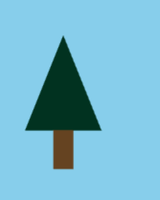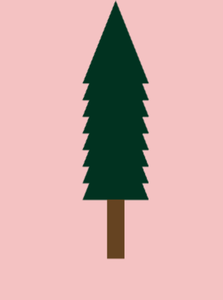Open in App
Not now

# Draw a tree using arcade library in Python

• Difficulty Level : Expert
• Last Updated : 15 Nov, 2021

Drawing a tree isn’t a tough task in Python using the turtle module. But, what if you can draw it using Arcade Module as well. Arcade is an object-oriented library. It can be installed like any other Python Package using an import arcade.

Approach:

• Define a function to draw trees. Here, we are drawing a pine tree made up of a rectangle and a triangle. So, you can use the inbuilt arcade function to rectangle and triangle.
```def draw_tree(x, y):

# Draw the triangle on top of the trunk
x, y - 100,
x + 80, y - 100,
# Draw the trunk
arcade.draw_lrtb_rectangle_filled(x + 30, x + 50, y - 100, y - 140,
• Now, since we have defined the function to tree, let’s define the main function and under which define arcade.open_window() to specify the screen width, height, and title. Also, use arcade.start_render() and arcade.finish_render to instruct arcade module when to start and stop drawing. Finally, add the arcade.run() to specify the ending.
```def main():
# Open the window

# Start the render process.

# Call our drawing functions.
draw_tree(50, 250)

# Finish the render.

# keep the window up .
main()```

Example 1:

## Python3

 `import` `arcade`  `def` `draw_tree(x, y):` `    ``# Draw the triangle on top of the trunk``    ``arcade.draw_triangle_filled(x ``+` `40``, y,``                                ``x, y ``-` `100``,``                                ``x ``+` `80``, y ``-` `100``,``                                ``arcade.color.DARK_GREEN)` `    ``# Draw the trunk``    ``arcade.draw_lrtb_rectangle_filled(x ``+` `30``, x ``+` `50``, y ``-` `100``, y ``-` `140``,``                                      ``arcade.color.DARK_BROWN)`  `def` `main():` `    ``# Open the window``    ``arcade.open_window(``600``, ``600``, ``"TREE"``)``    ``arcade.set_background_color(arcade.color.SKY_BLUE)` `    ``# Start the render process.``    ``arcade.start_render()` `    ``# Call our drawing functions.``    ``draw_tree(``50``, ``250``)` `    ``# Finish the render.``    ``arcade.finish_render()` `    ``# Keep the window up.``    ``arcade.run()`  `main()`

Output:Example 2:

## Python3

 `#import module``import` `arcade` `# specify spacing``Column_spacing ``=` `20``Row_spacing ``=` `20``Left_margin ``=` `110``Bottom_margin ``=` `400` `# Open the window and set the background``arcade.open_window(``700``, ``700``, ``"BOX"``)` `# set background color``arcade.set_background_color(arcade.color.BABY_PINK)` `# Start the render process. This must be done before any drawing commands.``arcade.start_render()` `# Loop for each row``for` `row ``in` `range``(``8``):``    ``# Loop for each column``    ``for` `column ``in` `range``(``8``):``        ``# Calculate our location``        ``x ``=` `column ``*` `Column_spacing ``+` `Left_margin``        ``y ``=` `row ``*` `Row_spacing ``+` `Bottom_margin` `        ``# Draw the item``    ``arcade.draw_triangle_filled(x ``+` `40``, y,``                                ``x, y ``-` `100``,``                                ``x ``+` `80``, y ``-` `100``,``                                ``arcade.color.DARK_GREEN)` `arcade.draw_lrtb_rectangle_filled(x ``+` `30``, x ``+` `50``, ``300``, ``230``,``                                      ``arcade.color.DARK_BROWN)` `# Finish the render.``arcade.finish_render()` `# Keep the window up until someone closes it.``arcade.run()` `# This code is contributed by pulkitagarwal03pulkit`

Output:-My Personal Notes arrow_drop_up